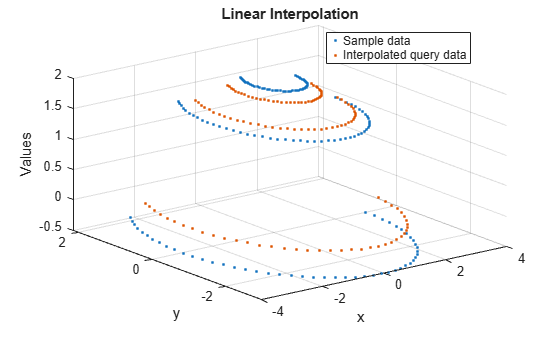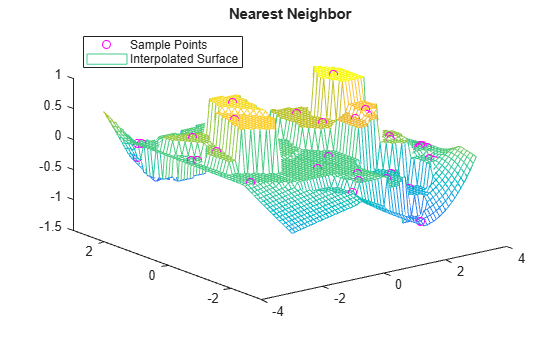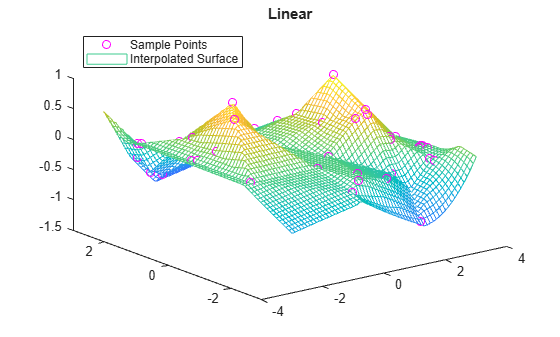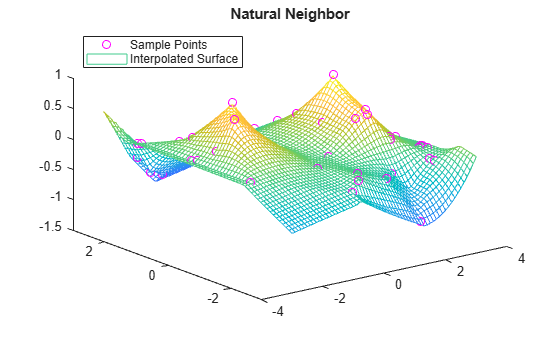Documentation

### This is machine translation

Mouseover text to see original. Click the button below to return to the English version of the page.

# scatteredInterpolant

Interpolate 2-D or 3-D scattered data

## Description

Use `scatteredInterpolant` to perform interpolation on a 2-D or 3-D data set of scattered data. `scatteredInterpolant` returns the interpolant `F` for the given data set. You can evaluate `F` at a set of query points, such as `(xq,yq)` in 2-D, to produce interpolated values `vq = F(xq,yq)`.

Use `griddedInterpolant` to perform interpolation with gridded data.

## Creation

### Syntax

``F = scatteredInterpolant``
``F = scatteredInterpolant(x,y,v)``
``F = scatteredInterpolant(x,y,z,v)``
``F = scatteredInterpolant(P,v)``
``F = scatteredInterpolant(___,Method)``
``F = scatteredInterpolant(___,Method,ExtrapolationMethod)``

### Description

````F = scatteredInterpolant` creates an empty scattered data interpolant object.```

example

````F = scatteredInterpolant(x,y,v)` creates an interpolant that fits a surface of the form v = F(x,y). Vectors `x` and `y` specify the `(x,y)` coordinates of the sample points. `v` is a vector that contains the sample values associated with the points `(x,y)`.```

example

````F = scatteredInterpolant(x,y,z,v)` creates a 3-D interpolant of the form v = F(x,y,z).```

example

````F = scatteredInterpolant(P,v)` specifies the coordinates of the sample points as an array. The rows of `P` contain the (x, y) or (x, y, z) coordinates for the values in `v`.```

example

````F = scatteredInterpolant(___,Method)` specifies an interpolation method: `'nearest'`, `'linear'`, or `'natural'`. Specify `Method` as the last input argument in any of the first three syntaxes.```

example

````F = scatteredInterpolant(___,Method,ExtrapolationMethod)` specifies both the interpolation and extrapolation methods. Pass `Method` and `ExtrapolationMethod` together as the last two input arguments in any of the first three syntaxes.`Method` can be: `'nearest'`, `'linear'`, or `'natural'`.`ExtrapolationMethod` can be: `'nearest'`, `'linear'`, or `'none'`.```

### Input Arguments

expand all

Sample points, specified as vectors of the same size as `v`. The sample points should be unique. However, if the sample points contain duplicates, `scatteredInterpolant` displays a warning and merges the duplicates into a single point.

Data Types: `double`

Sample points array, specified as an `m`-by-`n` matrix, where `m` is the number of points and `n` is the dimension of the space where the points reside. Each row of `P` contains the (x, y) or (x, y, z) coordinates of a sample point. The sample points should be unique. However, if the sample points contain duplicates, `scatteredInterpolant` displays a warning and merges the duplicates into a single point.

Data Types: `double`

Sample values, specified as a vector that defines the function values at the sample points, v = F(x,y,z).

Data Types: `double`

Interpolation method, specified as one of these options.

MethodDescriptionContinuity
`'linear'` (default)

Linear interpolation

C0
`'nearest'`

Nearest neighbor interpolation

Discontinuous
`'natural'`

Natural neighbor interpolation

C1 (except at sample points)

Extrapolation method, specified as one of these options.

ExtrapolationMethodDescription
`'linear'`

Linear extrapolation based on boundary gradients. Default when `Method` is `'linear'` or `'natural'`.

`'nearest'`

Nearest neighbor extrapolation. This method evaluates to the value of the nearest neighbor on the boundary. Default when `Method` is `'nearest'`.

`'none'`

No extrapolation. Any queries outside the convex hull of `Points` return `NaN`.

## Properties

expand all

Sample points, specified as a matrix. The size of the matrix is `m`-by-`2` or `m`-by-`3` to represent `m` points in 2-D or 3-D space. Each row of `Points` contains the (x, y) or (x, y, z) coordinates of a unique sample point. The rows in `Points` correspond to the function values in `Values`.

Data Types: `double`

Function values at sample points, specified as a vector of values associated with each point in `Points`.

Data Types: `double`

Interpolation method, specified as `'linear'`,`'nearest'` , or `'natural'`. See `Method` for descriptions of these methods.

Extrapolation method, specified as `'nearest'`, `'linear'`, or `'none'`. See `ExtrapolationMethod` for descriptions of these methods.

Data Types: `double`

## Usage

Use `scatteredInterpolant` to create the interpolant, `F`. Then you can evaluate `F` at specific points using any of the following syntaxes:

```Vq = F(Pq) Vq = F(Xq,Yq) Vq = F(Xq,Yq,Zq) Vq = F({xq,yq}) Vq = F({xq,yq,zq}) ```

• `Vq = F(Pq)` specifies the query points in the matrix `Pq`. Each row in `Pq` contains the coordinates of a query point.

• `Vq = F(Xq,Yq)` and `Vq = F(Xq,Yq,Zq)` specify the query points as two or three matrices of equal size.

• `Vq = F({xq,yq})` and `Vq = F({xq,yq,zq})` specify the query points as grid vectors. Use this syntax to conserve memory when you want to query a large grid of points.

## Examples

collapse all

Define some sample points and calculate the value of a trigonometric function at those locations. These points are the sample values for the interpolant.

```t = linspace(3/4*pi,2*pi,50)'; x = [3*cos(t); 2*cos(t); 0.7*cos(t)]; y = [3*sin(t); 2*sin(t); 0.7*sin(t)]; v = repelem([-0.5; 1.5; 2],length(t));```

Create the interpolant.

`F = scatteredInterpolant(x,y,v);`

Evaluate the interpolant at query locations (`xq`,`yq`).

```tq = linspace(3/4*pi+0.2,2*pi-0.2,40)'; xq = [2.8*cos(tq); 1.7*cos(tq); cos(tq)]; yq = [2.8*sin(tq); 1.7*sin(tq); sin(tq)]; vq = F(xq,yq);```

Plot the result.

```plot3(x,y,v,'.',xq,yq,vq,'.'), grid on title('Linear Interpolation') xlabel('x'), ylabel('y'), zlabel('Values') legend('Sample data','Interpolated query data','Location','Best')```Create an interpolant for a set of scattered sample points, then evaluate the interpolant at a set of 3-D query points.

Define 200 random points and sample a trigonometric function. These points are the sample values for the interpolant.

```P = -2.5 + 5*gallery('uniformdata',[200 3],0); v = sin(P(:,1).^2 + P(:,2).^2 + P(:,3).^2)./(P(:,1).^2+P(:,2).^2+P(:,3).^2);```

Create the interpolant.

`F = scatteredInterpolant(P,v);`

Evaluate the interpolant at query locations (`xq`,`yq`,`zq`).

```[xq,yq,zq] = meshgrid(-2:0.25:2); vq = F(xq,yq,zq);```

Plot slices of the result.

```xslice = [-.5,1,2]; yslice = [0,2]; zslice = [-2,0]; slice(xq,yq,zq,vq,xslice,yslice,zslice)```Replace the elements in the `Values` property when you want to change the values at the sample points. You get immediate results when you evaluate the new interpolant because the original triangulation does not change.

Create 50 random points and sample an exponential function. These points are the sample values for the interpolant.

```x = -2.5 + 5*gallery('uniformdata',[50 1],0); y = -2.5 + 5*gallery('uniformdata',[50 1],1); v = x.*exp(-x.^2-y.^2);```

Create the interpolant.

`F = scatteredInterpolant(x,y,v)`
```F = scatteredInterpolant with properties: Points: [50x2 double] Values: [50x1 double] Method: 'linear' ExtrapolationMethod: 'linear' ```

Evaluate the interpolant at `(1.40,1.90)`.

`F(1.40,1.90)`
```ans = 0.0029 ```

Change the interpolant sample values and reevaluate the interpolant at the same point.

```vnew = x.^2 + y.^2; F.Values = vnew; F(1.40,1.90)```
```ans = 6.1109 ```

Compare the results of several different interpolation algorithms offered by `scatteredInterpolant`.

Create a sample data set of 50 scattered points. The number of points is artificially small to highlight the differences between the interpolation methods.

```x = -3 + 6*rand(50,1); y = -3 + 6*rand(50,1); v = sin(x).^4 .* cos(y);```

Create the interpolant and a grid of query points.

```F = scatteredInterpolant(x,y,v); [xq,yq] = meshgrid(-3:0.1:3);```

Plot the results using the `'nearest'`, `'linear'`, and `'natural'` methods. Each time the interpolation method changes, you need to requery the interpolant to get the updated results.

```F.Method = 'nearest'; vq1 = F(xq,yq); plot3(x,y,v,'mo') hold on mesh(xq,yq,vq1) title('Nearest Neighbor') legend('Sample Points','Interpolated Surface','Location','NorthWest')``````F.Method = 'linear'; vq2 = F(xq,yq); figure plot3(x,y,v,'mo') hold on mesh(xq,yq,vq2) title('Linear') legend('Sample Points','Interpolated Surface','Location','NorthWest')``````F.Method = 'natural'; vq3 = F(xq,yq); figure plot3(x,y,v,'mo') hold on mesh(xq,yq,vq3) title('Natural Neighbor') legend('Sample Points','Interpolated Surface','Location','NorthWest')```Plot the exact solution.

```figure plot3(x,y,v,'mo') hold on mesh(xq,yq,sin(xq).^4 .* cos(yq)) title('Exact Solution') legend('Sample Points','Exact Surface','Location','NorthWest')```Query an interpolant at a single point outside the convex hull using nearest neighbor extrapolation.

Define a matrix of 200 random points and sample an exponential function. These points are the sample values for the interpolant.

```P = -2.5 + 5*gallery('uniformdata',[200 2],0); x = P(:,1); y = P(:,2); v = x.*exp(-x.^2-y.^2);```

Create the interpolant, specifying linear interpolation and nearest neighbor extrapolation.

`F = scatteredInterpolant(P,v,'linear','nearest')`
```F = scatteredInterpolant with properties: Points: [200x2 double] Values: [200x1 double] Method: 'linear' ExtrapolationMethod: 'nearest' ```

Evaluate the interpolant outside the convex hull.

`vq = F(3.0,-1.5)`
```vq = 0.0031 ```

Disable extrapolation and evaluate `F` at the same point.

```F.ExtrapolationMethod = 'none'; vq = F(3.0,-1.5)```
```vq = NaN ```

expand all

## Tips

• It is quicker to evaluate a `scatteredInterpolant` object `F` at many different sets of query points than it is to compute the interpolations separately using the functions `griddata` or `griddatan`. For example:

```% Fast to create interpolant F and evaluate multiple times F = scatteredInterpolant(X,Y,V) v1 = F(Xq1,Yq1) v2 = F(Xq2,Yq2) % Slower to compute interpolations separately using griddata v1 = griddata(X,Y,V,Xq1,Yq1) v2 = griddata(X,Y,V,Xq2,Yq2) ```
• To change the interpolation sample values or interpolation method, it is more efficient to update the properties of the interpolant object `F` than it is to create a new `scatteredInterpolant` object. When you update `Values` or `Method`, the underlying Delaunay triangulation of the input data does not change, so you can compute new results quickly.

## Algorithms

`scatteredInterpolant` uses a Delaunay triangulation of the scattered sample points to perform interpolation .

 Amidror, Isaac. “Scattered data interpolation methods for electronic imaging systems: a survey.” Journal of Electronic Imaging. Vol. 11, No. 2, April 2002, pp. 157–176.